# Looking Outward, From Inside a Black Hole

[Written by J. E. Tohline, early morning of 13 October 2017]  The relationship between the mass,$~M$, and radius,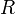$~R$, of a black hole is,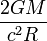$~\frac{2GM}{c^2 R}$$~=$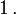$~1 \, .$

The mean density of matter inside a black hole of mass$~M$ is, therefore,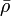$~\bar\rho$$~=$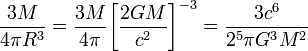$~ \frac{3M}{4\pi R^3} = \frac{3M}{4\pi} \biggl[ \frac{2GM}{c^2}\biggr]^{-3} = \frac{3c^6}{2^5\pi G^3 M^2}$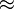$~\approx$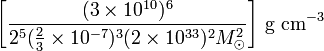$~ \biggl[\frac{(3 \times 10^{10})^6}{2^5 (\tfrac{2}{3}\times 10^{-7})^3 (2\times 10^{33})^2 M_\odot^2}\biggr] ~\mathrm{g}~\mathrm{cm}^{-3}$$~\approx$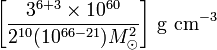$~ \biggl[\frac{3^{6+3} \times 10^{60}}{2^{10} (10^{66-21}) M_\odot^2}\biggr] ~\mathrm{g}~\mathrm{cm}^{-3}$$~\approx$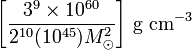$~ \biggl[\frac{3^{9} \times 10^{60}}{2^{10} (10^{45}) M_\odot^2}\biggr] ~\mathrm{g}~\mathrm{cm}^{-3}$$~\approx$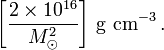$~ \biggl[\frac{2 \times 10^{16}}{M_\odot^2}\biggr] ~\mathrm{g}~\mathrm{cm}^{-3} \, .$

We are accustomed to imagining that the interior of a black hole (BH) must be an exotic environment because a one solar-mass BH has a mean density that is on the order of, but larger than, the density of nuclear matter. From the above expression, however, we see that a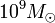$~10^9 M_\odot$ BH has a mean density that is less than that of water (1 gm/cm3). And the mean density of a BH having the mass of the entire universe must be very small indeed. This leads us to the following list of questions.

## Enumerated Questions

1. Can we construct a Newtonian structure out of normal matter that has a mass of, say,$~10^9 M_\odot$ whose equilibrium radius is much less than the radius of the BH horizon associated with that object? Does it necessarily have a mean temperature whose associated sound speed is super-relativistic?
2. Who else in the published literature has explored questions along these lines?